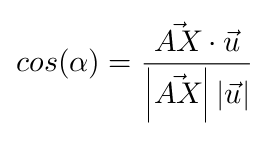# Algebra Description of Infinite cone

Ben shared this question 4 years ago

Hi, I was wondering if someone could tell me how the equation of the infinite cone is found from the input data <point> <vector> <angle> when using the InfiniteCone command.

Thanks!1

try the formula of cos of angle: ((X-A) · u)/(|X-A|*|u|)=cos(alpha) then calculate the square of the equation for a cuadric

[X=(x,y,z)]1

How does this relate to the <point> <vector> <angle> input data? I can guess that <alpha> = angle, but is u = <vector>(axis of cone)? What are X and A?1

X generic point (x,y,z) variables for the equation

A vertex of cone

u vector direction of cone

alpha anglethen do power 2 for eliminate sqrt() of denominator